JEE  >  VITEEE Maths Test - 6

# VITEEE Maths Test - 6

Test Description

## 40 Questions MCQ Test VITEEE: Subject Wise and Full Length MOCK Tests | VITEEE Maths Test - 6

VITEEE Maths Test - 6 for JEE 2023 is part of VITEEE: Subject Wise and Full Length MOCK Tests preparation. The VITEEE Maths Test - 6 questions and answers have been prepared according to the JEE exam syllabus.The VITEEE Maths Test - 6 MCQs are made for JEE 2023 Exam. Find important definitions, questions, notes, meanings, examples, exercises, MCQs and online tests for VITEEE Maths Test - 6 below.
Solutions of VITEEE Maths Test - 6 questions in English are available as part of our VITEEE: Subject Wise and Full Length MOCK Tests for JEE & VITEEE Maths Test - 6 solutions in Hindi for VITEEE: Subject Wise and Full Length MOCK Tests course. Download more important topics, notes, lectures and mock test series for JEE Exam by signing up for free. Attempt VITEEE Maths Test - 6 | 40 questions in 50 minutes | Mock test for JEE preparation | Free important questions MCQ to study VITEEE: Subject Wise and Full Length MOCK Tests for JEE Exam | Download free PDF with solutions
 1 Crore+ students have signed up on EduRev. Have you?
VITEEE Maths Test - 6 - Question 1

### The equation of the tangent to the curve (1 + x2)y = 2 - x where it crosses x-axis is

VITEEE Maths Test - 6 - Question 2

### The area bounded by the parabola x = 4 - y2 and the y-axis in square units, is

VITEEE Maths Test - 6 - Question 3

### If z (2 - i)= 3 + i,z20 =

VITEEE Maths Test - 6 - Question 4

If x2 + x + 1 is a factor of ax3 + bx2 + cx +d,then the real root of ax3 + bx2 + cx + d = 0

VITEEE Maths Test - 6 - Question 5

If f: R → R is defined by f x = x − [ x ] , where [x] is the greatest integer not exceeding x, then the set of discontinuities of f is

VITEEE Maths Test - 6 - Question 6

The area bounded by the curve y=xsinx and x-axis between x=0 and x=2π is

VITEEE Maths Test - 6 - Question 7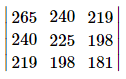VITEEE Maths Test - 6 - Question 8

The value of determinant ∆ of 3rd order is 9 then the value of ∆′2 where ∆′ is a determinant formed by cofactors of the element of ∆ is

VITEEE Maths Test - 6 - Question 9

If the function f is defined by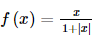then at what points is f differentiable

VITEEE Maths Test - 6 - Question 10

Integrating factor of differential equation cos x dy/dx+y sin x = 1 is

VITEEE Maths Test - 6 - Question 11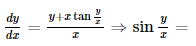VITEEE Maths Test - 6 - Question 12

The number of solutions of dy/dx = (y+1)/(x-1) when y (1) = 2 is :

Detailed Solution for VITEEE Maths Test - 6 - Question 12VITEEE Maths Test - 6 - Question 13

The solution of the equation ydx+(1+x2)tan-1xdy=0 is

VITEEE Maths Test - 6 - Question 14

If S is the set of all real x such that 2x - 1/2x3 + 3x2 + x is positive, then S contains

VITEEE Maths Test - 6 - Question 15

The value of ∫ sin−1 (cos x) dx is equal to

VITEEE Maths Test - 6 - Question 16

(d/dx)[tan-1((cosx-sinx)/(cosx+sinx))]=

VITEEE Maths Test - 6 - Question 17

∫ x2exx=

VITEEE Maths Test - 6 - Question 18

If cos-1p + cos-1q+cos-1r = π, then p2 + q2 + r2 + 2pqr is equal to

Detailed Solution for VITEEE Maths Test - 6 - Question 18

Let cos-1 p = α, cos-1 q = β and cos-1 r = γ
⇒ cosα = p, cosβ = q and cosγ = r
From question, α + β + γ = π
∴ cos (α + β) = cos (π - γ)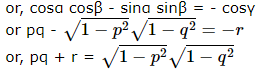Squaring, we get p2q2 + r2 + 2pqr = 1 - p2 - q2 + p2q2
or, p2 + q2 + r2 + 2pqr = 1

VITEEE Maths Test - 6 - Question 19

If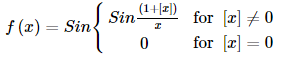for [ x ] = 0 where [x] denotes the greatest integer not exceeding x, then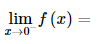VITEEE Maths Test - 6 - Question 20

The value of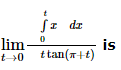VITEEE Maths Test - 6 - Question 21

Which of the following is not a statement in logic ?
1. Earth is planet .
2. Plants are living object .
3.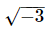is rational number .
4. x2 - 5x + 6 < 0, where x ∈ − R

VITEEE Maths Test - 6 - Question 22

If A is a square matrix of order 3 x 3 , then adj (adj A) is equal to

VITEEE Maths Test - 6 - Question 23

If A is singular matrix of order n, then a (adj A) equals,

VITEEE Maths Test - 6 - Question 24

P,Q,R and S have to deliver lecture, then in how many ways can the lectures be arranged?

Detailed Solution for VITEEE Maths Test - 6 - Question 24

Total no. of ways delivering lectures = 4P4 = 4! = 24

VITEEE Maths Test - 6 - Question 25
The chance of getting a doublet with 2 dice is
VITEEE Maths Test - 6 - Question 26

When two balls are drawn from a bag containing 2 white, 4 red and 6 black balls, the chance for both of them to be red is

VITEEE Maths Test - 6 - Question 27
Let E₁,E₂, E₃ be three arbitrary events of a sample space S consider the following statements which of the following statement is correct?
VITEEE Maths Test - 6 - Question 28

If in ∆ABC ∠A=450, ∠C=600, then a+c√2=

VITEEE Maths Test - 6 - Question 29

The nth term of the series 3, √3, 1,... is 1/243, then n is

VITEEE Maths Test - 6 - Question 30

The co-efficient of correlation between two variables x and y is 0.5, their covariance is 16. If the S.D. of x is 4, then the S.D. of y is equal to

Detailed Solution for VITEEE Maths Test - 6 - Question 30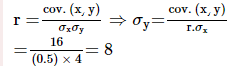VITEEE Maths Test - 6 - Question 31

The quartile deviation of daily wages (in Rs.) of 7 persons given below:
12, 7, 15, 10, 17, 17, 25 is

VITEEE Maths Test - 6 - Question 32

The roots of the equation (q-r)x2+(r-p)x+(p-q)=0 are

VITEEE Maths Test - 6 - Question 33

Roots of the equation 5x2-7x+k=0 are reciprocal of each other, then the value of k is

VITEEE Maths Test - 6 - Question 34

A line makes the same angle θ, with each of the x and z axis. If the angle β, which it makes with y-axis, is such that sin2β = 3sin2θ, then cos2θ equals:

VITEEE Maths Test - 6 - Question 35

The shortest distance between the lines (x - 3)/3 = (y - 8)/-1 = (z - 3)/1 and (x + 3)/-3 = (y + 7)/2 = (z -6)/4 is

VITEEE Maths Test - 6 - Question 36

The general solution of the equation tan2θ.tanθ=1 for n∈I is, θ is equal to

VITEEE Maths Test - 6 - Question 37

A circle touches the x-axis and also touches the cirlce with centre at (0, 3) and radius 2. The locus of the centre of the cirlce is

VITEEE Maths Test - 6 - Question 38

The locus of a point whose abscissa and ordinate are always equal is

VITEEE Maths Test - 6 - Question 39

If |a|=3, |b|=4, |c|=5 and a+b+c=0, the angle between a and b is

VITEEE Maths Test - 6 - Question 40

If vectors 3i+2j-k and 6i-4xj+yk are parallel, then the value of x and y are

## VITEEE: Subject Wise and Full Length MOCK Tests

1 videos|3 docs|73 tests
 Use Code STAYHOME200 and get INR 200 additional OFF Use Coupon Code
Information about VITEEE Maths Test - 6 Page
In this test you can find the Exam questions for VITEEE Maths Test - 6 solved & explained in the simplest way possible. Besides giving Questions and answers for VITEEE Maths Test - 6, EduRev gives you an ample number of Online tests for practice

## VITEEE: Subject Wise and Full Length MOCK Tests

1 videos|3 docs|73 tests